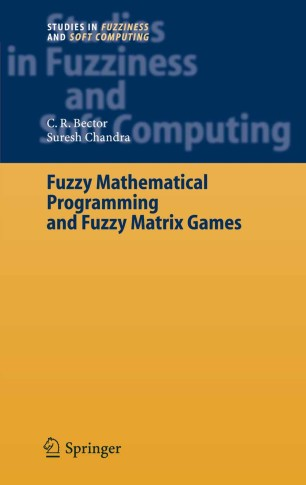# Fuzzy Mathematical Programming and Fuzzy Matrix Games

• C. R. Bector
• Suresh ChandraBook

Part of the Studies in Fuzziness and Soft Computing book series (STUDFUZZ, volume 169)

1. Front Matter
Pages I-XII
2. Pages 21-38
3. Pages 39-56
4. Pages 117-132
5. Pages 133-155
6. Pages 157-174
7. Pages 175-198
8. Back Matter
Pages 230-236

### Introduction

This book presents a systematic and focused study of the application of fuzzy sets to two basic areas of decision theory, namely Mathematical Programming and Matrix Game Theory. Apart from presenting most of the basic results available in the literature on these topics, the emphasis is on understanding their natural relationship in a fuzzy environment. The study of duality theory for fuzzy mathematical programming problems plays a key role in understanding this interrelationship. For this, a theoretical framework of duality in fuzzy mathematical programming and conceptualization of the solution of a fuzzy game is created on the lines of their crisp counterparts. Most of the theoretical results and associated algorithms are illustrated through small numerical examples from actual applications.

### Keywords

Arithmetic Duality Fuzzy Fuzzy Arithmetic Fuzzy Games Fuzzy Linear Programming Fuzzy Matrix Games Fuzzy Optimization algorithm algorithms decision theory fuzzy set game theory optimization programming

#### Authors and affiliations

• C. R. Bector
• 1
• Suresh Chandra
• 2